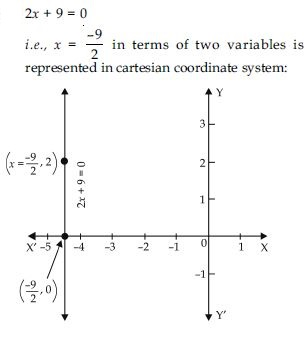# NCERT Solutions for Class 9 Math Chapter 4 - Linear Equation in two Variables

##### Question 1:

The cost of a notebook is twice the cost of a pen. Write a linear equation in two variables to represent this statement. (Take the cost of a notebook to be `x and that of a pen to be `y)

Let the cost of a note book is `x and cost of a pen is `y.
Therefore a linear equation in two variables (x and y) representing the given statement is x = 2y.

##### Question 2:

Express the following linear equation in the form ax + by + c = 0 and indicate the values of a, b and c in each case.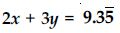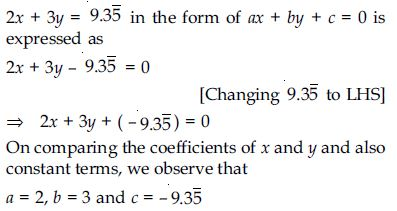##### Question 3:

Express the following linear equation in the form ax + by + c = 0 and indicate the values of a, b and c in each case.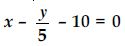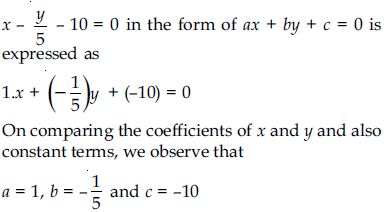##### Question 4:

Express the following linear equation in the form ax + by + c = 0 and indicate the values of a, b and c in each case.
– 2x + 3y = 6

–2x + 3y = 6 in the form of ax + by + c = 0 is
written as
–2x + 3y – 6 = 0 [Changing 6 to LHS]
$⇒$ – 2x + 3y + (–6) = 0
On comparing the coefficients of x and y and also constant terms, we observe that
a = –2, b = 3 and c = –6

##### Question 5:

Express the following linear equation in the form ax + by + c = 0 and indicate the values of a, b and c in each case.
x = 3y

x = 3y in the form of ax + by + c = 0 is written as
x – 3y = 0
$⇒$ 1.x + (–3)y + 0 = 0
On comparing the coefficients of x and y and also constant terms, we observe that
a = 1, b = – 3 and c = 0.

##### Question 6:

Express the following linear equation in the form ax + by + c = 0 and indicate the values of a, b and c in each case.
2x = – 5y

2x = –5y in the form of
ax + by + c = 0 is written as 2x + 5y = 0
[Changing –5y to LHS]
$⇒$ 2x + 5y + 0 = 0
On comparing the coefficients of x and y and also constant terms, we observe that
a = 2, b = 5 and c = 0

##### Question 7:

Express the following linear equation in the form ax + by + c = 0 and indicate the values of a, b and c in each case.
3x + 2 = 0

3x + 2 = 0 in the form of ax + by + c = 0 is written
as
3x + 0.y + 2 = 0
On comparing the coefficients of x and y and also constant terms, we observe that
a = 3, b = 0 and c = 2.

##### Question 8:

Express the following linear equation in the form ax + by + c = 0 and indicate the values of a, b and c in each case.
y – 2 = 0

y – 2 = 0 in the form of ax + by + c = 0 is written as
0.x + 1.y + (–2) = 0
On comparing the coefficients of x and y and also
constant terms, we observe that
a = 0, b = 1 and c = –2.

##### Question 9:

Express the following linear equation in the form ax + by + c = 0 and indicate the values of a, b and c in each case.
5 = 2x

5 = 2x in the form of ax + by + c = 0 is written as
5 – 2x = 0
$⇒$ –2x + 0.y + 5 = 0
On comparing the coefficients of x and y also constant terms, we observe that
a = –2, b = 0 and c = 5

##### Question 10:

Which one of the following option is true, and why? y = 3x + 5 has
(i) a unique solution
(ii) only two solutions
(iii) infinitely many solutions.

Statement (iii) is true because for every value of x, there is corresponding value of y and vice-versa. Thorough justification:
Let assume that
(i) x = 0 then y = 3 × 0 + 5
$⇒$ y = 0 + 5
$⇒$ y = 5
So, x = 0, y = 5 is a solution.
(ii) x = 1; then y = 3 × 1 + 5
$⇒$ y = 3 + 5
$⇒$ y = 8
So, x = 1, y = 8 is also a solution.
(iii) x = –2 then y = 3 (–2) + 5
$⇒$ y = –6 + 5
$⇒$ y = –1
So, x = –2, y = –1 is also a solution.
Similarly, we can find infinitely many solutions of given equation as we wish simply by putting the value of x (or y) and get the corresponding value of y (or x).

##### Question 11:

Write four solutions for each of the following equations:
2x + y = 7

2x + y = 7
We can write equation as:
y = 7 – 2x
When x = 0;
y = 7 – 2 × 0 $⇒$ y = 7 – 0 $⇒$ y = 7
When x = 1;
y = 7 – 2 × 1 $⇒$ y = 7 – 2 $⇒$ y = 5
When x = 2;
y = 7 – 2 × 2 $⇒$ y = 7 – 4 $⇒$ y = 3
When x = –1;
y = 7 – 2 × (–1) $⇒$ y = 7 + 2 $⇒$ y = 9
So, four of the infinitely many solutions of the equation 2x + y = 7 are (0, 7), (1, 5), (2, 3) and (–1, 9).

##### Question 12:

Write four solutions for each of the following equations:
$\mathrm{\pi }$x + y = 9

$\mathrm{\pi }$x + y = 9
We can write the equation as:
y = 9 – $\mathrm{\pi }$x
When x = 0;
y = 9 – $\mathrm{\pi }$ 0 $⇒$ y = 9 – 0 $⇒$ y = 9
When x = 2;
y = 9 – $\mathrm{\pi }$ 2 $⇒$ y = 9 – 2$\mathrm{\pi }$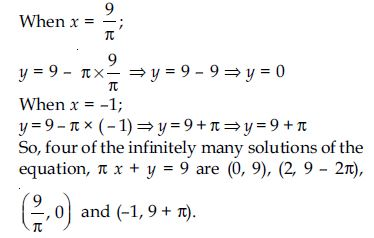##### Question 13:

Write four solutions for each of the following equations:
x = 4y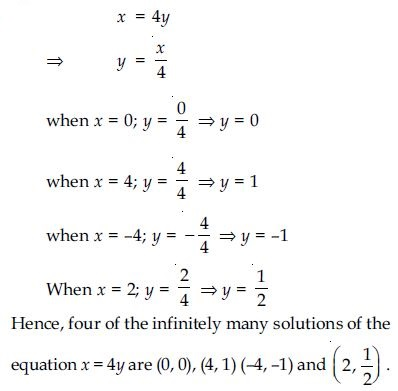##### Question 14:

Check which of the following are solutions of the equation x – 2y = 4 and which are not?
(0, 2)

Put x = 0 and y = 2 in equation
x – 2y = 4
LHS, x – 2y = 0 – 2 × 2
= 0 – 4 = –4
RHS = 4
Clearly, LHS $\ne$ RHS
$\therefore$ (0, 2) is not the solution of equation
x – 2y = 4.

##### Question 15:

Check which of the following are solutions of the equation x – 2y = 4 and which are not?
(2, 0)

Put x = 2 and y = 0 in equation
x – 2y = 4
LHS, x – 2y = 2 – 2 × 0
= 2 – 0 = 2
RHS = 4
Clearly LHS $\ne$ RHS
$\therefore$ (2, 0) is not the solution of x – 2y = 4.

##### Question 16:

Check which of the following are solutions of the equation x – 2y = 4 and which are not?
(4, 0)

Put x = 4 and y = 0 in the equation
x – 2y = 4
LHS, x – 2y = 4 – 2 × 0 = 4 – 0 = 4
RHS = 4
Clearly, LHS = RHS
$\therefore$ (4, 0) is the solution of equation x – 2y = 4.

##### Question 17:

Check which of the following are solutions of the equation x – 2y = 4 and which are not?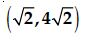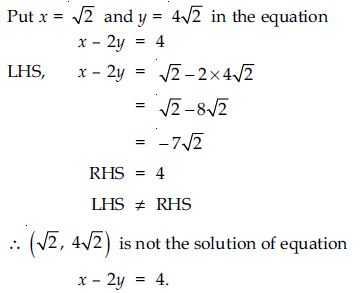##### Question 18:

Check which of the following are solutions of the equation x – 2y = 4 and which are not?
(1, 1)

Put x = 1, y = 1 in the equation x – 2y = 4
LHS, x – 2y = 1 – 2 × 1 = 1 – 2 = – 1
RHS = 4
Clearly, LHS $\ne$ RHS
$\therefore$ (1, 1) is not the solution of equation
x – 2y = 4.

##### Question 19:

Find the value of k, if x = 2, y = 1 is a solution of the equation 2x + 3y = k.

If x = 2, y = 1 is the solution of equation 2x + 3y = k, then it definitely satisfy the equation.
$\therefore$ 2 × 2 + 3 × 1 = k
(Substituting x = 2, y = 1 in the equation)
$⇒$ 4 + 3 = k
$⇒$ 7 = k
or k = 7
Hence, required value of k is 7.

##### Question 20:

Draw the graph of each of the following linear equations in two variables:
x + y = 4

x + y = 4 $⇒$ y = 4 – x
Table showing the values of y corresponding to the values of x is as follows: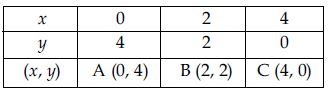Plot the points A(0, 4), B(2, 2) and C(4, 0) on a
graph paper. Join these points and obtain a line.
Scale chosen:
On X-axis: 5 divisions = 1 unit
On Y-axis: 5 divisions = 1 unit.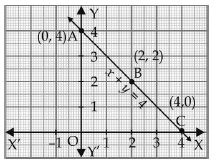##### Question 21:

Draw the graph of each of the following linear equations in two variables:
x – y = 2

x – y = 2 $⇒$ – y = 2 – x
or y = x – 2
Table showing the values of y corresponding
to the values of x is as follows: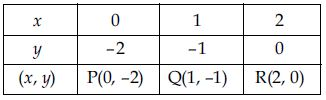Plot the points P(0, –2), Q(1, –1) and R(2, 0) on a graph paper. Join these points and obtain a line.
Scale chosen:
On X-axis: 5 divisions = 1 unit
On Y-axis: 5 divisions = 1 unit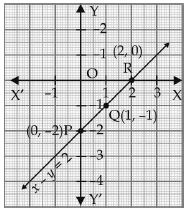##### Question 22:

Draw the graph of each of the following linear equations in two variables:
y = 3x

y = 3x
Table showing the values of y corresponding to the values of x is as follows: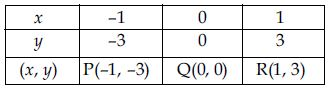Plot the points P(–1, –3), Q(0, 0) and R(1, 3) on a graph paper. Join these points and obtain a line.
Scale chosen:
On X-axis: 5 divisions = 1 unit
On Y-axis: 5 divisions = 1 unit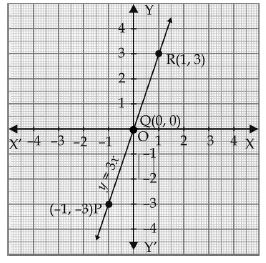##### Question 23:

Draw the graph of each of the following linear equations in two variables:
3 = 2x + y

3= 2x + y
$⇒$ 3 – 2x = y
or y = 3 – 2x
Table showing the values of y corresponding to the values of x is as follows: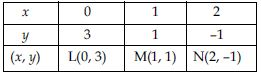Plot the points L(0, 3), M(1, 1) and N(2, –1) on a graph paper. Join these points and obtain a line.
Scale chosen:
On X-axis: 5 divisions = 1 unit
On Y-axis: 5 divisions = 1 unit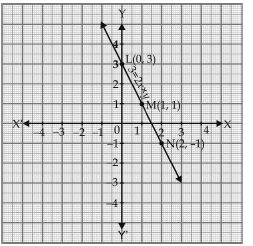##### Question 24:

Give the equations of two lines passing through (2, 14). How many more such lines are there, and why?

As we know that through a point, infinitely many lines can be drawn. So out of these; any two linear equations which are satisfied by the point (2, 14) are as follows:
Let ax + by = c be linear equation in x and y which is satisfied by the point (2, 14).
It means (2, 14) lie on it:
$\therefore$ 2a + 14b = c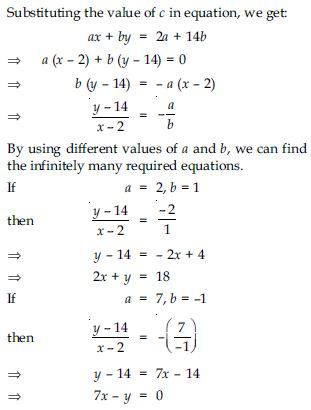##### Question 25:

If the point (3, 4) lies on the graph of the equation 3y = ax + 7, Find the value of a.

Given that point (3, 4) lie on the graph corresponding to:
3y = ax + 7
So, it satisfy this linear equation.
There by
3 × 4 = a × 3 + 7
$⇒$ 12 = 3a + 7
$⇒$ 12 – 7 = 3a
$⇒$ 5 = 3a
or 3a = 5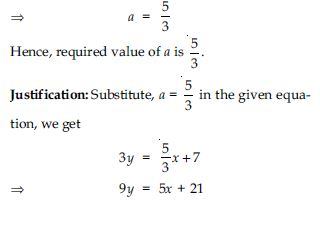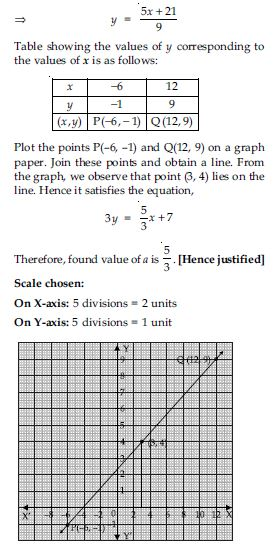##### Question 26:

The taxi fare in a city is as follows: For the first kilometre, the fare is `8 and for the subsequent distance it is `5 per kilometre. Taking the distance covered as x km and total fare as `y, write a linear equation for this information, and draw its graph.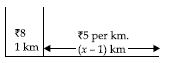Taking total distance travelled as x km.
For first kilometer, fare = `8
For subsequent distance i.e., for remaining distance;
fare per kilometer = `5
$\therefore$ Fare for remaining (x – 1) km
= `5 (x – 1)
Total fare = `y
$\therefore$ `8 + `5(x – 1) = `y
$⇒$ 8 + 5(x – 1) = y
$⇒$ 8 + 5x – 5 = y
$⇒$ 5x + 3 = y
$⇒$ 5x – y + 3 = 0 ...(1)
Which is a linear equation for the given information. To plot the graph of equation (1) the table showing the value of y corresponding to the values
of x is as follows: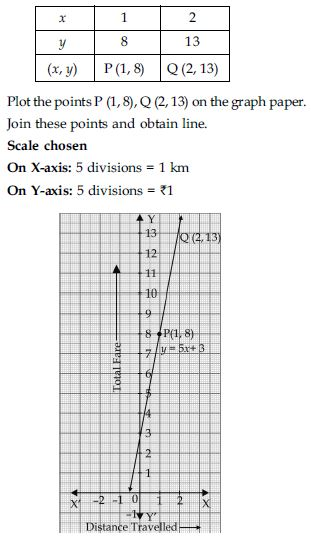##### Question 27:

From the choices given below, choose the equation whose graphs are given in Fig. (a) and Fig. (b).
For fig. (a)    For fig. (b)
(i) y = x    (i) y = x + 2
(ii) x + y = 0    (ii) y = x – 2
(iii) y = 2x    (iii) y = – x + 2
(iv) 2 + 3y = 7x    (iv) x + 2y = 6.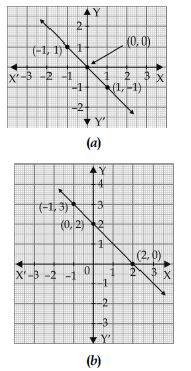Every point on the graph (a) is satisfied in the equation; (ii) x + y = 0
Therefore, for graph (a) the correct equation out of four equations is:
x + y = 0
$⇒$ y = –x
For x = 0, y = 0
For x = 1, y = –1
For x = –1, y = 1
Now, every point on the graph (b) is satisfied in the equation (iii) y = – x + 2
Therefore, for graph (b) the correct equation out of four equations is:
y = –x + 2
For x = 2,
y = – 2 + 2
$⇒$ y = 0
For x = 0,
y = – 0 + 2
$⇒$ y = 2
For x = –1,
y = – (–1) + 2
$⇒$ y = 1 +
$⇒$ y = 3

##### Question 28:

If the work done by a body on application of a constant force is directly proportional to the distance travelled by the body, express this in the form of an equation in two variables and draw the graph of the same by taking the constant force as 5 units. Also read from the graph the work done when the distance travelled by the body is:
(i) 2 units (ii) 0 unit.

Let work done by constant force (say k units) is y units and distance travelled by the body is x units. As we know that work done by a force is directly proportional to the distance travelled by the body. From ratio and proportion; we can express this fact as:
y = kx
Taking k = 5 units
$\therefore$ y = 5x ...(1)
Which is a linear equation in two variables.
To plot the graph of equation (1); the table showing the value of y corresponding to the values of x is as follows: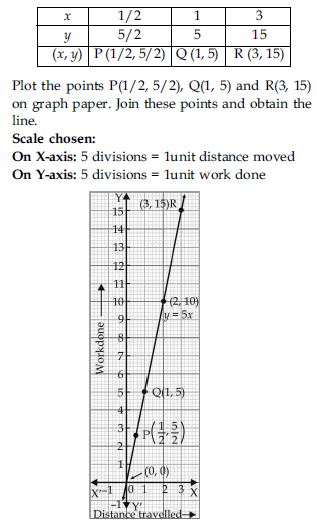From the graph, we observe that when
(i) x = 2 units
then y = 10 units
Hence, when distance moved by the body is 2 units then work done is 10 units.
(ii) When x = 0 units
then y = 0 units
Hence, when no distance is moved by the body then no work is done on body.

##### Question 29:

Yamini and Fatima, two students of Class IX of a school, together contributed `100 towards the Prime Minister’s Relief Fund to help the earthquake victims. Write a linear equation which satisfies this data. (You may take their contributions as `x and `y.) Draw the graph of the same.

Let Yamini contributed `x and Fatima contributed `y towards Prime Minister’s Relief Fund. Contribution of both = `100
$⇒$ x + y = 100 ...(1)
which is a linear equation in two variables.
To plot the graph of equation (1); we draw the table showing the values of y corresponding to the values of x.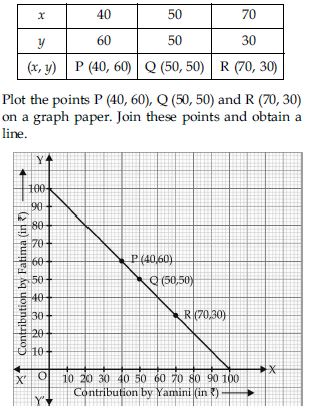##### Question 30:

In countries like USA and Canada, temperature is measured in Fahrenheit, whereas in countries like India, it is measured in Celsius. Here is a linear equation that converts Fahrenheit to Celsius: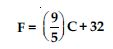Draw the graph of above linear equation above using Celsius for x-axis and Fahrenheit for y-axis.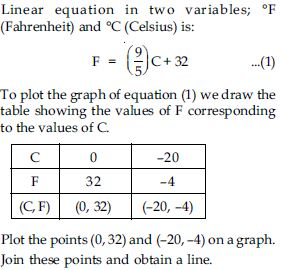##### Question 31:

In countries like USA and Canada, temperature is measured in Fahrenheit, whereas in countries like India, it is measured in Celsius. Here is a linear equation that converts Fahrenheit to Celsius:If the temperature is 30$°$C, what is the temperature in Fahrenheit?

From the graph; if temperature is 30$°$C, then temperature in Fahrenheit is 86$°$F.

##### Question 32:

In countries like USA and Canada, temperature is measured in Fahrenheit, whereas in countries like India, it is measured in Celsius. Here is a linear equation that converts Fahrenheit to Celsius:If the temperature is 95$°$F, what is the temperature in Celsius?

From the graph; if temperature is 95$°$F then temperature in celsius is 35$°$C.

##### Question 33:

In countries like USA and Canada, temperature is measured in Fahrenheit, whereas in countries like India, it is measured in Celsius. Here is a linear equation that converts Fahrenheit to Celsius:If the temperature is 0$°$C, what is the temperature in Fahrenheit and if the temperature is 0$°$F, what is the temperature in Celsius?

From the graph; if temperature is 0$°$C then temperature in Fahrenheit is 32$°$F. If temperature is 0$°$F, then temperature in Celsius is –17.8$°$C (approximately).

##### Question 34:

In countries like USA and Canada, temperature is measured in Fahrenheit, whereas in countries like India, it is measured in Celsius. Here is a linear equation that converts Fahrenheit to Celsius:Is there a temperature which is numerically the same in both Fahrenheit and Celsius? If yes, find it?

Yes; there is a temperature which is numerically same in both Fahrenheit and Celsius. From the graph we observe that point (–40, –40) lie on line.
Hence, –40$°$ (both in F and C) is numerically the same temperature.
(Note: – 40$°$F $\ne$ – 40$°$C).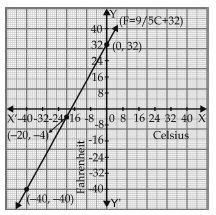##### Question 35:

Give the geometric representation of y = 3 as an equation in one variable

y = 3 in terms of one variable is represented on a number line.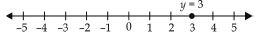##### Question 36:

Give the geometric representation of y = 3 as an equation in two variables.

y = 3 in terms of two variables is represented in cartesian coordinate system.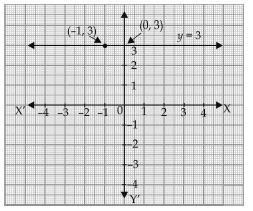##### Question 37:

Give the geometric representation of 2x + 9 = 0 as an equation in one variable

2x + 9 = 0 in terms of one variable is represented on number line:
2x + 9 = 0
$⇒$ 2x = –9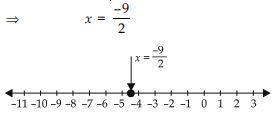##### Question 38:

Give the geometric representation of 2x + 9 = 0 as an equation in two variables